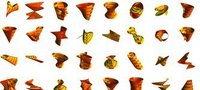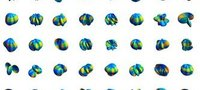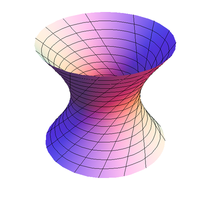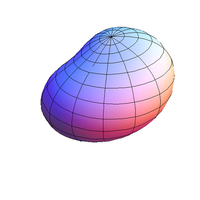6760, Math 21a, Fall 2009
Exhibits page Math 21a 2009, Multivariable Calculus
Ruled surfaces
Office: SciCtr 434

## Problem A: Ruled Surface and bumpy sphere

 Problem A: (creative assignment). Submit online your own ruled surface as well as your own bumpy sphere. The examples should be different from the examples given. Try to build some examples which look good. We will make an exhibit with the best submissions. SUBMIT. Only submit the formulas for f[t],g[t],rho[t]. You do not need to include the ParametricPlot part.Gallery of ruled surfaces 2009 Gallery of bumpy spheres 2009 Asteroid project 2007 Marble book project 2006Ruled surfaceBumpy sphere Your ruled surface and a bumpy sphere can be entered here in this poll. Please use Mathematica notation, where functions are capitalized and square brackets are used in arguments. ```f[t_]:={Cos[t],Sin[t],0}; g[t_]:= {Cos[t+2],Sin[t+2],2}; ``` We will exhibit the best submissions. Be creative! What is a ruled surface? The vector valued functions f[t] and g[t] form two closed curves in space. The ruled surface is obtained by connecting corresponding points on these curves with lines. You can use either a flash applet from this source or use Mathematica. Here is example code for a ruled surface in Mathematica ```f[t_]:={Cos[t],Sin[t],0}; g[t_]:= {Cos[t+2],Sin[t+2],2}; ParametricPlot3D[s f[t] +(1-s) g[t],{t,0,2Pi},{s,0,1}] ``` And here is an example of a bumpy sphere in Mathematica ```rho[t_,s_]:=(4+Sin[s] Sin[2 s + t]); ParametricPlot3D[rho[t,s] {Sin[s] Cos[t],Sin[s] Sin[t],Cos[s]},{s,0,Pi},{t,0,2Pi}] ``` which is equivalent to ```rho[t_,s_]:=(4+Sin[s] Sin[2 s + t]); SphericalPlot3D[rho[t,s],{s, 0, Pi},{t,0,2Pi}] ``` In a bumpy sphere, the radius rho depends on the Euler angles theta (abbreviated t) and phi (abbreviated s).